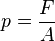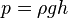# Pressure

Pressure (symbol "p") is the force applied to a surface (in a direction perpendicular to that surface) per unit area of the surface. If the force is concentrated on a small area, it will exert higher pressure than if the same force is distributed over a larger surface area. For example, a force applied with a finger may be able to push a thumbtack into a wall, but the same finger pressing directly against the wall (with the same force) may not make any lasting impression. This is because the thumbtack concentrates the force into a smaller area.

Our understanding of the concept of pressure and our ability to manipulate the pressure in various systems have provided us with many practical benefits. For example, we have been able analyze weather patterns, move fluids using pumps, sterilize medical equipment at temperatures above the boiling point of water, and conduct industrial processes at reduced temperatures.

Pressure arises from two fundamentally different kinds of sources: ambient and localized. Ambient sources of pressure are usually a gas or a liquid in which an entity is immersed, such as a human being on the surface of the earth or an octopus in the sea. Life forms are generally insensitive to ambient pressures and become aware of the source of that pressure when currents become strong enough that the fluid exerts a non-uniform localized pressure on the life form, such as when the wind blows. Humans also become sensitive to ambient pressure during the take off and landing of passenger airlines when they need to swallow to equalize the air pressure on both sides of the ear drum.

Localized pressure sources are usually discrete objects, such as the finger pressing on the wall, or the tires of a car pressed against the pavement. A liquid or gas can become the source of a localized pressure if either of them is forced through a narrow opening.

Flight by birds and airplanes occurs according to simple principles of pressure whereby the faster a fluid moves in one direction the lesser is the pressure it exerts in the direction perpendicular to its flow. The wings of birds and airplanes are shaped in a way that their movement through the air requires a faster air flow over the top of the wing than over the bottom, which means that the upward force produced by the air flowing under the wing is greater than the downward force produced by the air flowing over the wing. The result is lift which pushes the bird or airplane upward.

## Mathematical expression

In mathematical terms, pressure can be expressed as:$p = \frac{F}{A}\,$

where:$p$ is the pressure$F$ is the normal force$A$ is the area.

The SI unit for force is the Newton, that for area is square meter, and that for pressure is called a pascal (Pa). By definition, 1 Pa = 1 Newton per square meter (N/m2). [1 Pa is also equivalent to 1 Joule per cubic meter (J•m−3) or 1 kilogram per meter per square second (kg•m−1•s−2).] The units for pressure are further discussed below.

## Kinetic nature of pressure in a gas

In 1738, Swiss physician and mathematician Daniel Bernoulli (1700-1782) published Hydrodynamica, which laid the basis for the kinetic theory of gases. In this work, Bernoulli made the argument, still used to this day, that gases consist of great numbers of molecules moving in all directions, and that their impact on a surface causes the gas pressure that we feel. In addition, he proposed that what we experience as heat is simply the kinetic energy of their motion.

## Relative pressure or gauge pressure

For gases, pressure is sometimes measured not as an absolute pressure, but relative to atmospheric pressure. Such a measurement is sometimes called gauge pressure. For example, if the air pressure in an automobile tire is given as "220 kPa," it is actually 220 kPa above atmospheric pressure. Given that atmospheric pressure at sea level is about 100 kPa, the absolute pressure in the tire is therefore about 320 kPa. In technical work, this is written as "a gauge pressure of 220 kPa." Where space is limited, such as on pressure gauges, name plates, graph labels, and table headings, the use of a modifier in parentheses, such as "kPa (gauge)" or "kPa (absolute)," is permitted. In non-SI technical work, gauge pressure is sometimes written as "32 psig (pounds per square inch gauge)."

## Scalar nature of pressure

The pressure within a fluid (gas or liquid) is a scalar quantity—that is, it has magnitude but no particular direction associated with it in space. When the fluid meets a solid surface, this pressure is transmitted to the solid in a direction perpendicular to that surface. For instance, a swimmer at the bottom of a swimming pool may find that no matter how he turns his head (while maintaining the same depth), the water pressure against his eardrums remains the same, and it seems to be perpendicular to the eardrums.

A gas is said to be static if it as a whole does not appear to move. The individual molecules of the gas, however, are in constant random motion. Given that there is an extremely large number of molecules and the motion of the individual molecules is random in every direction, we do not detect any motion. If we enclose the gas within a container, the molecules collide with the walls of the container and exert pressure against the walls. The pressure at any point within the gas is independent of direction.

## Negative pressure

Although pressure generally has a positive value, it may have a negative value in some situations. Some examples follow.

• A relative (gauge) pressure can be negative. For instance, an absolute pressure of 80 kPa may be described as a gauge pressure of -21 kPa (that is, 21 kPa below atmospheric pressure).
• At times, attractive forces (such as Van der Waals forces) between the particles of a fluid exceed repulsive forces. Such scenarios are generally unstable, because the particles will move closer together until repulsive forces balance attractive forces.
• Negative pressure exists in the transpiration pull of plants.

## Stagnation pressure

Stagnation pressure is the pressure a fluid exerts when it is forced to stop moving. Consequently, although a fluid moving at higher speed will have a lower static pressure, it may have a higher stagnation pressure when forced to a standstill. Static pressure and stagnation pressure are related by the Mach number of the fluid. In addition, there can be differences in pressure due to differences in the elevation (height) of the fluid.

The pressure of a moving fluid can be measured using a Pitot probe (or one of its variations) connected to a manometer. Depending on where the inlet holes are located on the probe, it can measure static pressure or stagnation pressure.

## The pressure/volume conjugate pair

Conjugate variables
of thermodynamics
Pressure Volume
Temperature Entropy
Chem. potential Particle no.

In thermodynamics, pressure and volume are said to form a pair of conjugate variables (for nonviscous fluids). The pressure acts as a generalized force—pressure differences force a change in volume, and their product is the energy lost by the system due to mechanical work. Pressure is the driving force, and volume is the associated displacement.

## Calculation of hydrostatic pressure

At a given spot within a fluid, the hydrostatic pressure (or "head pressure") is the pressure resulting from the weight of the fluid column above that spot. It may be calculated by the following formula:$p = \rho g h\,$
where:
• ρ (rho) is the density of the fluid (for example, the density of freshwater is about 1000 kg/m3);
• g is the acceleration due to gravity (approximately 9.81 m/s2 on Earth's surface);
• h is the height of the fluid column (in meters).

## Units

The name for the unit of pressure, the (Pascal), was added in 1971. Before that, pressure in SI was expressed by using its units (N•m-2).

Non-SI measures (still in use in some parts of the world) include the pound-force per square inch (psi) and the bar.

The cgs unit of pressure is the barye (ba), which is equal to one dyne per square centimeter (dyn•cm-2).

The standard atmosphere (atm) of pressure is an established constant. It is approximately equal to typical air pressure at earth mean sea level and is defined as follows:

standard atmosphere = 101,325 Pa = 101.325 kPa = 1013.25 hPa.

Some meteorologists prefer the hectopascal (hPa) for atmospheric air pressure, which is equivalent to the older unit millibar (mbar). Similar pressures are given in kilopascals (kPa) in practically all other fields, where the hecto prefix is hardly ever used. In Canadian weather reports, the normal unit is kPa.

Because pressure may be measured by the displacement of a column of liquid in a manometer, it is sometimes expressed as the depth (in inches) of a particular fluid. The most common choices of fluid are mercury (Hg) and water, and pressure was once expressed in terms of inches of mercury (in Hg). Water is nontoxic and readily available, but mercury's density allows for a shorter column (and so a smaller manometer) to measure a given pressure. The pressure exerted by a column of liquid of height h and density ρ is given by the hydrostatic pressure equation noted above: p = hgρ.

Fluid density and local gravity can vary from one reading to another depending on local factors, so the height of a fluid column does not define pressure precisely. When 'millimeters of mercury' or 'inches of mercury' are quoted today, these units are not based on a physical column of mercury; rather, they have been given precise definitions that can be expressed in terms of SI units. (The water-based units still depend on the density of water, a measured, rather than defined, quantity.)

Although no longer favored in physics, these manometric units are still encountered in certain fields. Blood pressure is measured in millimeters of mercury in most parts of the world, and lung pressure in centimeters of water is also common. Natural gas pipeline pressures are measured in inches of water, expressed as WC ('Water Column'). Scuba divers often use a manometric rule of thumb: the pressure exerted by ten meters depth of water is approximately equal to one atmosphere.

Pressure is still sometimes expressed in kgf/cm² or grams-force/cm² (sometimes as kg/cm² and g/cm² without properly identifying the force units). But using the terms kilogram, gram, kilogram-force, or gram-force (or their symbols) as a unit of force is expressly forbidden in SI. (The unit of force in SI is the newton, N).

Non-SI units presently or formerly in use include the following:

• atmosphere.
• manometric units:
• centimeter, inch, and millimeter of mercury (Torr).
• millimeter, centimeter, meter, inch, and foot of water.
• imperial units:
• kip, ton-force (short), ton-force (long), pound-force, ounce-force, and poundal per square inch.
• pound-force, ton-force (short), and ton-force (long) per square foot.
• non-SI metric units:
• bar, millibar.
• kilogram-force, or kilopond, per square centimeter (technical atmosphere) (symbol: at) is 1 kgf/cm².
• gram-force and tonne-force (metric ton-force) per square centimeter.
• barye (dyne per square centimeter).
• kilogram-force and tonne-force (metric ton-force) per square meter.
• sthene per square meter (pieze).